Next: Three-Dimensional Wave Equation Up: Multi-Dimensional Waves Previous: Multi-Dimensional Waves

# Plane Waves

As we saw in the previous chapter, a sinusoidal wave of amplitude, wavenumber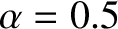, and angular frequency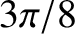, propagating in the positive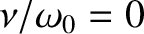-direction, can be represented in terms of a wavefunction of the form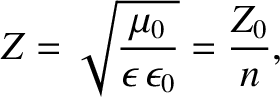(529)

This type of wave is conventionally termed a one-dimensional plane wave. It is one-dimensional because its associated wavefunction only depends on a single Cartesian coordinate. Furthermore, it is a plane wave because the wave maxima, which are located at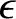(530)

where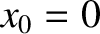is an integer, consist of a series of parallel planes, normal to the-axis, that are equally spaced a distanceapart, and propagate along the-axis at the fixed phase velocity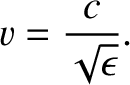, where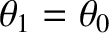is the characteristic wave speed. These conclusions follow because Equation (530) can be re-written in the form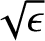(531)

where. Moreover, (531) is the equation of a plane, normal to the-axis, whose distance of closest approach to the origin is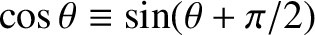.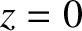The previous equation can also be written in the coordinate-free form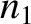(532)

where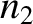is a unit vector directed along the-axis, andrepresents the vector displacement of a general point from the origin. Since there is nothing special about the-direction, it follows that ifis re-interpreted as a unit vector pointing in an arbitrary direction then Equation (532) can be re-interpreted as the general equation of a plane (Fitzpatrick 2008). As before, the plane is normal to, and its distance of closest approach to the origin is. (See Figure 38.) This observation allows us to write the three-dimensional equivalent to the wavefunction (529) as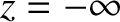(533)

where the constant vector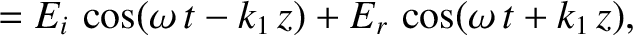is known as the wavevector. The wave represented in the previous expression is conventionally termed a three-dimensional plane wave. It is three-dimensional because its wavefunction,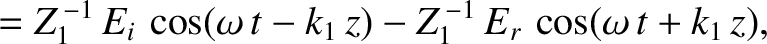, depends on all three Cartesian coordinates. Moreover, it is a plane wave because the wave maxima are located at(534)

or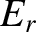(535)

where, and. The wavenumber,, is the magnitude of the wavevector,. That is,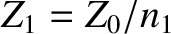. It follows, by comparison with Equation (532), that the wave maxima consist of a series of parallel planes, normal to the wavevector, that are equally spaced a distanceapart, and propagate in the-direction at the fixed phase velocity. (See Figure 39.) Hence, the direction of the wavevector corresponds to the direction of wave propagation. The most general expression for the wavefunction of a three-dimensional plane wave is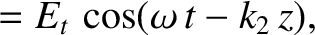, whereis a constant phase angle. As is readily demonstrated, the inclusion of a non-zero phase angle in the wavefunction shifts all the wave maxima a distance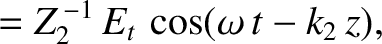in the-direction. In the following, whenever possible,is set to zero, for the sake of simplicity.Next: Three-Dimensional Wave Equation Up: Multi-Dimensional Waves Previous: Multi-Dimensional Waves
Richard Fitzpatrick 2013-04-08